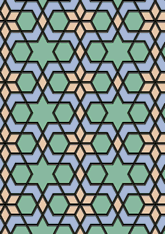# Eski Mosque, Edirnedata192/TUR0126

## Geometry

• The symmetry group of the tiling is *2222 (pmm).
• All the internal angles of the constituent polygons are a multiple of 60°.
• Contains five regular two-pointed star polygons with vertex angle of 60°.
• Contains two regular hexagons.
• Contains one regular 6-pointed star polygon with vertex angle of 60°.
• There is one non-regular reflective tile.
• The tiling does not satisfy the two-colour condition.
• The tiling is edge-to-edge.
• As drawn, contains about 309 polygons.

## References

Publications referenced: